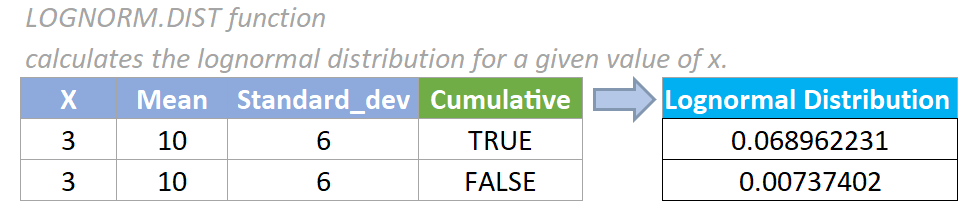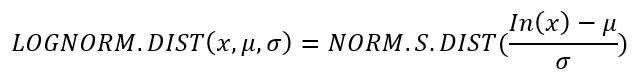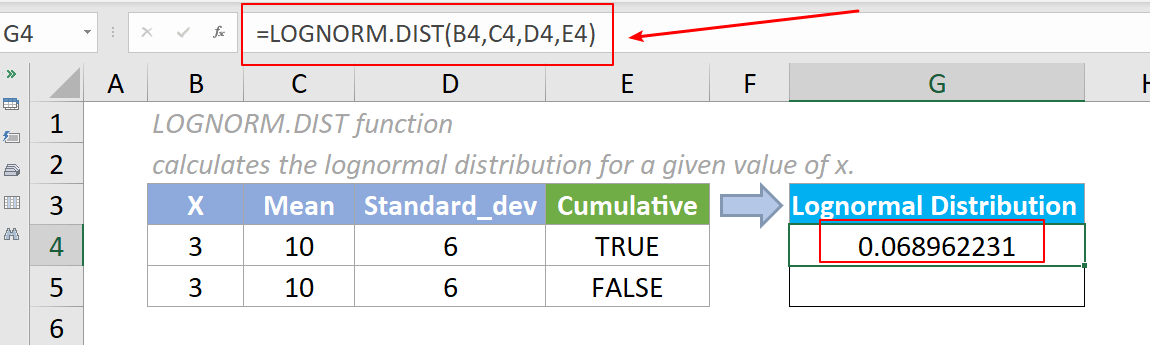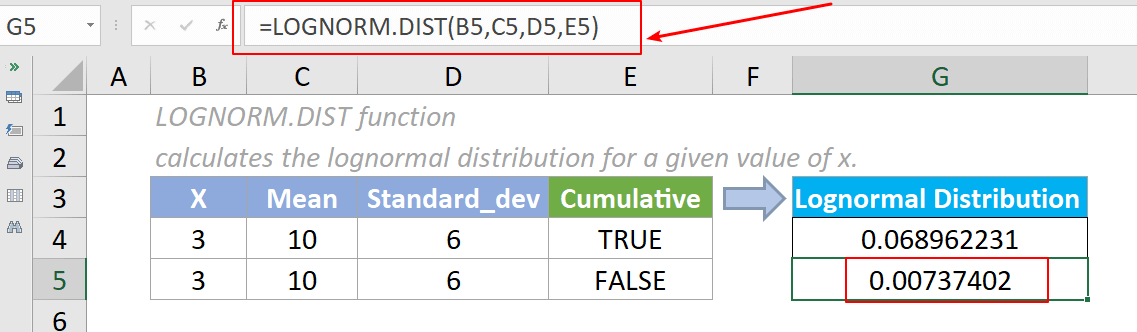## Excel LOGNORM.DIST Function

The LOGNORM.DIST function calculates the lognormal distribution for a given value of x. We can use this function to analyze data that has been logarithmically transformed.#### Syntax

=LOGNORM.DIST(x,mean,standard_dev,cumulative)

#### Arguments

• X (required): The value at which you want to evaluate the lognormal distribution.
• Mean (required): The arithmetic value of the mean of ln(x).
• Standard_dev (required): The value of the standard deviation of ln(x).
• Cumulative (required): A logical value specifying the type of distribution to be calculated. It is either a TRUE or FALSE value.
If it is TRUE, LOGNORM.DIST returns the cumulative distribution function.
If it is FLASE, LOGNORM.DIST returns the probability density function.

#### Return Value

The LOGNORM.DIST function returns a numeric value.

#### Function notes

1. The LOGNORM.DIST function was newly introduced In Excel 2010, so it is unavailable in earlier versions.
2. The #VALUE! error value occurs if any of the supplied arguments is non-numeric.
3. The #NUM! error value occurs if any of the below situation occurs:
• The supplied x is ≤ 0;
• The supplied standard_dev ≤ 0.
4. The equation for the hypergeometric distribution is:Where μ is the mean of ln(x) and σ is the standard deviation of ln(x).

#### Example

As the below screenshot shows, there lists the values of x, mean, standard_dev, and cumulative parameters, to get the cumulative lognormal distribution and probability lognormal distribution, please do as follows:

To calculate the cumulative lognormal distribution, please copy the formula below into cell G4, then press the Enter key to get the result.

=LOGNORM.DIST(B4,C4,D4,E4)

To calculate the cumulative lognormal distribution, please copy the formula below into cell G4, then press the Enter key to get the result.To calculate the probability lognormal distribution, please copy the formula below into cell G5, then press the Enter key to get the result.

=LOGNORM.DIST(B5,C5,D5,E5)Note: We can also directly input values in the formula. For example, the formula in cell G4 can be changed to:

=LOGNORM.DIST(3,10,6,TRUE)

#### Relative Functions:

• Excel EVEN Function
The EVEN function rounds numbers away from zero to the nearest even integer.

• Excel EXP Function
The EXP function returns the result of the constant e raised to the nth power.